#2018 iT 邦幫忙鐵人賽DAY 14
1
Software Development

# 枚舉 (Enumerations)

## enum 介紹

enum 定義了一組相關值的通用類型，並讓你能夠在代碼中以類型安全的方式處理這些值。

enum 在 Swift 語言中相較於其他語言更加靈活了，而且他不必為 enum 中每種 case 都提供值。如果我們為 enum 每個 case 都提供值，則該值可以是String，Character 或任何 Int 或是 Float-point 類型的值。

Swift 中的枚舉是具有自己權限的 first-class 類型，他們採用許多慣例上只被 class 中的支援的方式，像是計算屬性提供有關 enum 目前值的附加訊息，而且實例方法提供提供 enum 表示值有關聯的功能。枚舉也可以定義初始器來提供初始 case 中的值；並可以擴大到超出原本能夠實現範圍的功能，並且符合協議以提供標準功能。

## enum 語法

``````enum EnumerationName { // 輸入 enum 名稱
//在此定義 enum 的 case
}
``````

``````enum Direction {
case up
case down
case left
case right
}
``````

``````enum Letter {
case A, B, C, D, E
case F, G, H, I, J
}
``````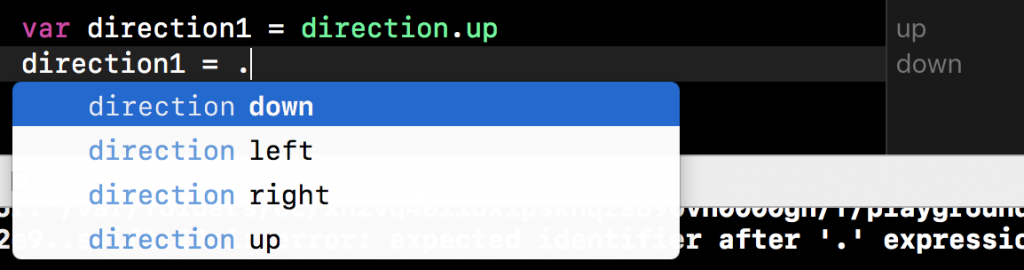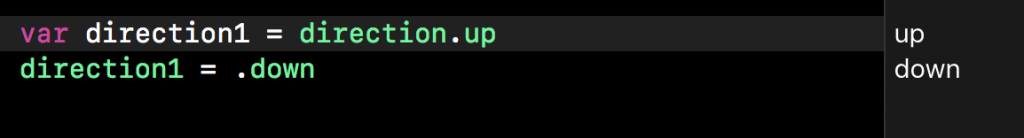## 使用 Switch 語句來匹配 enum 值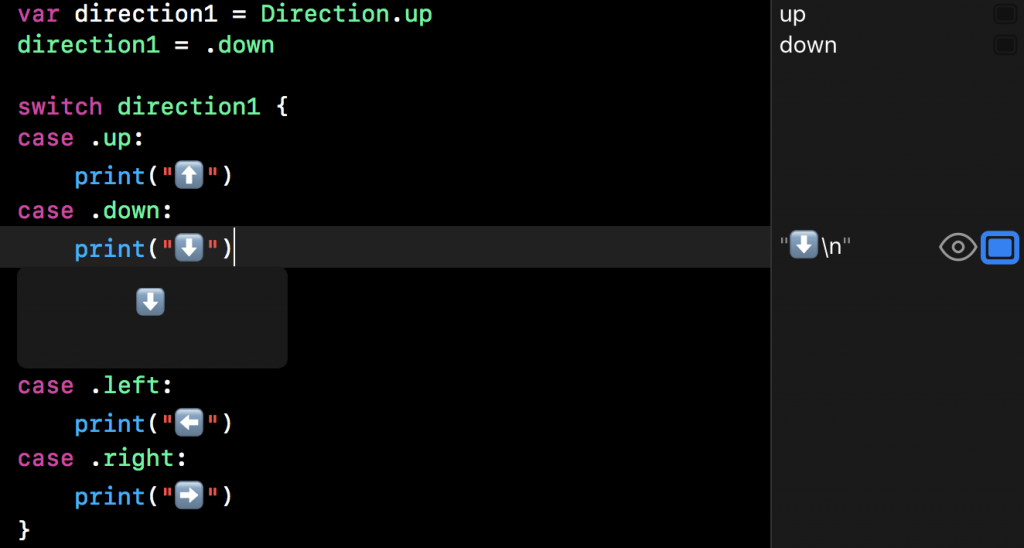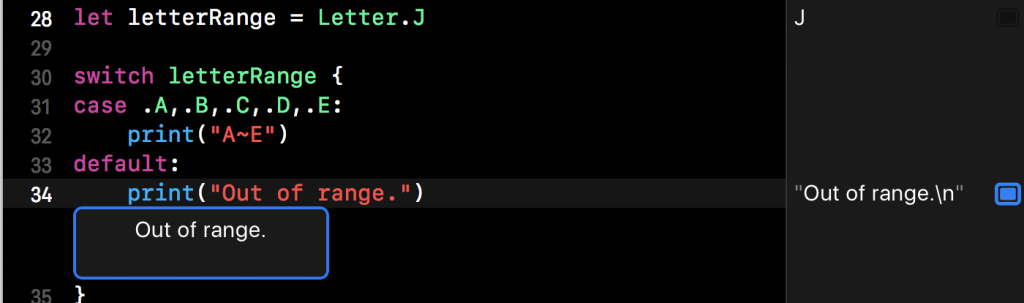## 關聯值 (Associated Values)

``````enum Code {
case numCode(Int, Int, Int, Int) //由四個 Int 值所組成
case nameCode(String,Int) //由一個 String 及一個 Int 值組成
}
``````

``````var productCode = Code.nameCode("蘋果", 20)
``````

``````productCode = .numCode(830, 775, 08, 20)
``````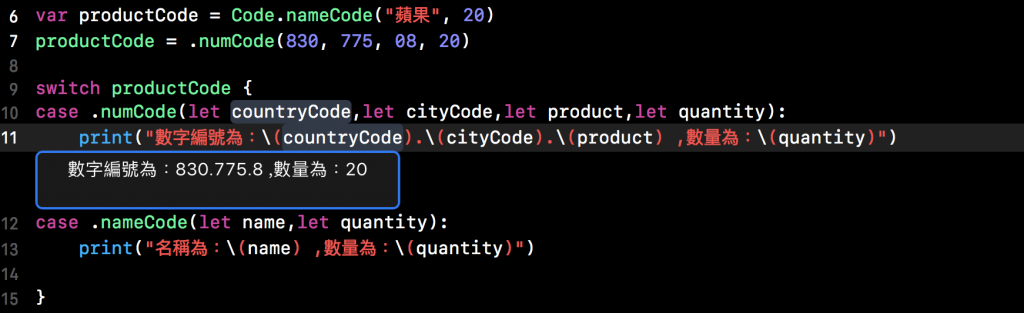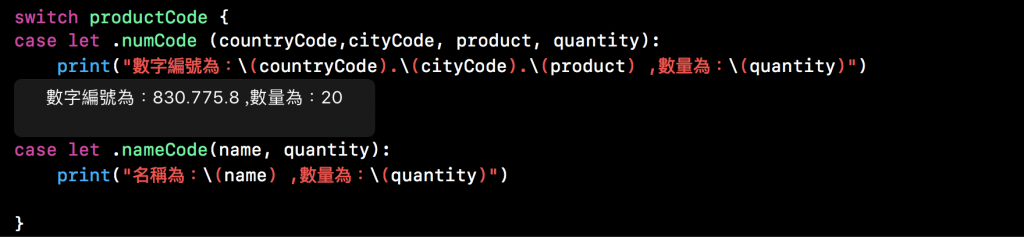# 原始值 (Raw Values)

``````enum numEnglish:Int {
case one = 1
case two = 2
case three = 3
}
``````

# 隱式指定的原始值 (Implicitly Assigned Raw Values)

``````enum AlphabeticalOrder:Int {
case A = 1, B , C , D ,F ,G
}
``````

``````enum Direction:String {
case up, down, left, right
}
``````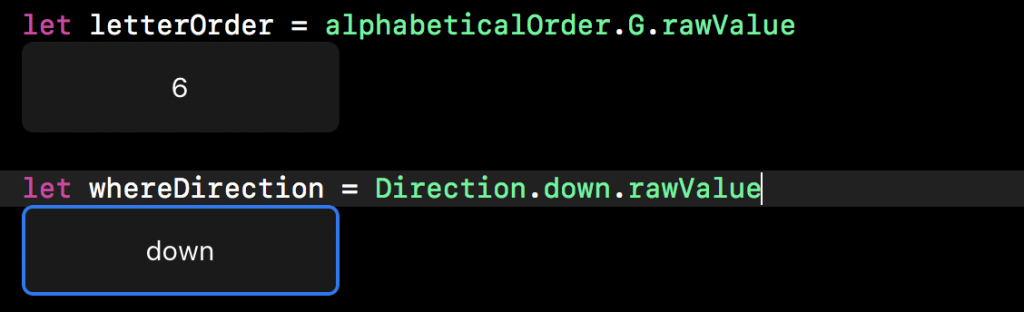# 從原始值初始化 (Initializing from a Raw Value)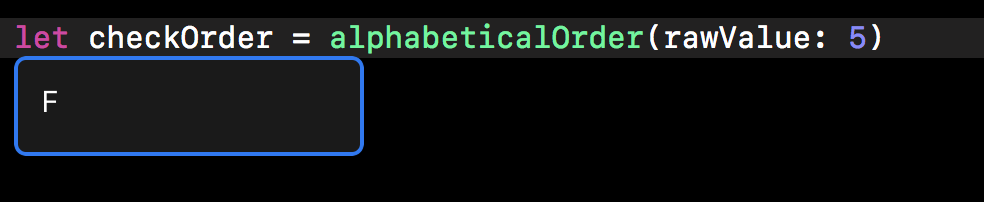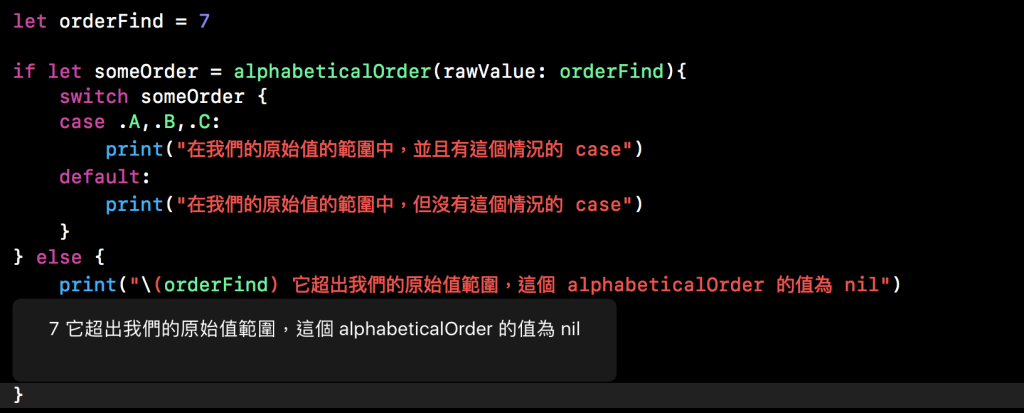# 遞歸枚舉 (Recursive Enumerations)

``````enum Calculation {
case number(Int)
indirect case add(Calculation,Calculation)
indirect case sub(Calculation,Calculation)
}
``````

``````indirect enum Calculation {
case number(Int)
case add(Calculation,Calculation)
case sub(Calculation,Calculation)
}
``````

``````let five = Calculation.number(5)
let two = Calculation.number(2)
let sum = Calculation.add(five , two)
let product = Calculation.mul(sum, Calculation.number(2))
``````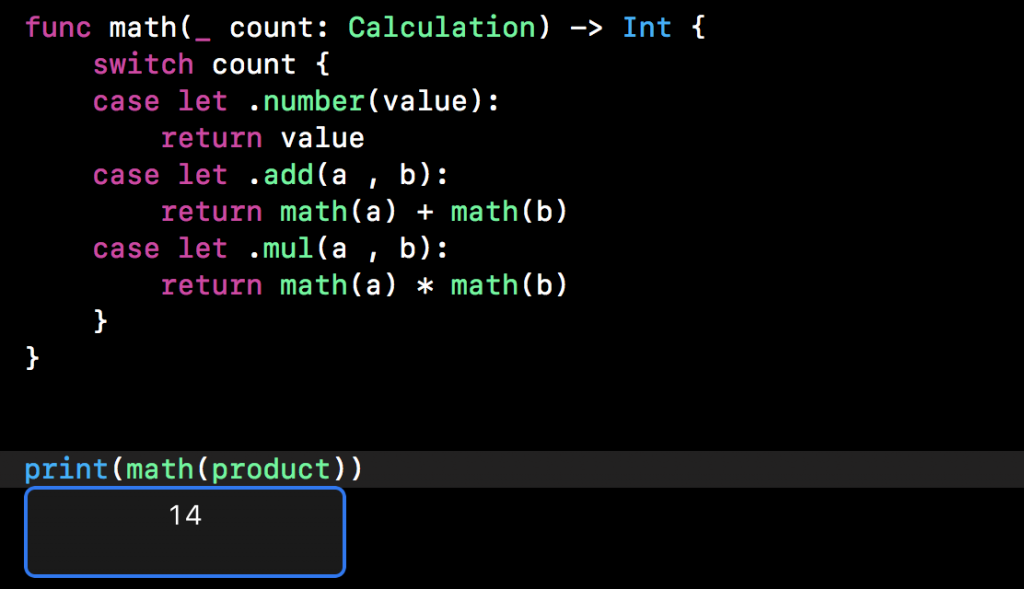# 補充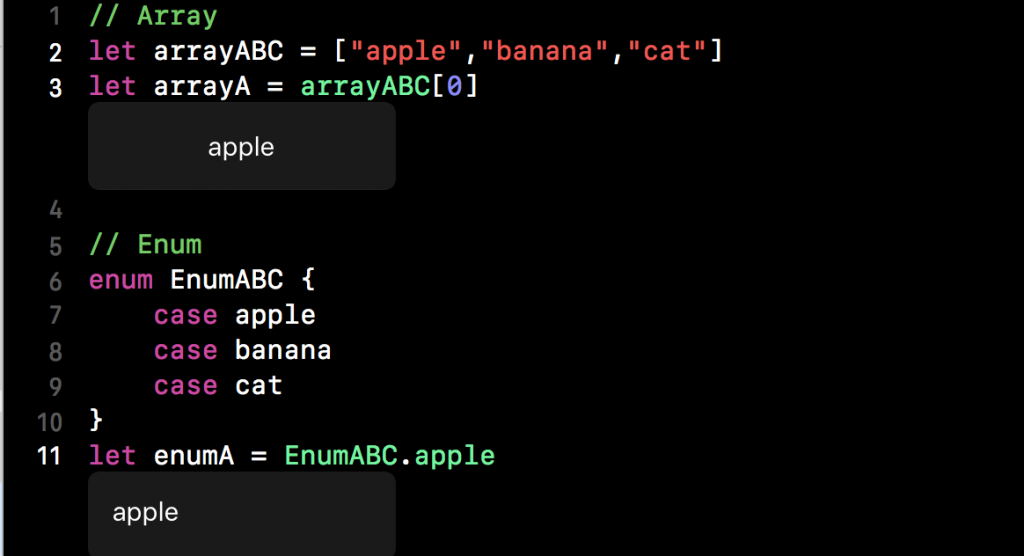Swift 菜鳥的30天30